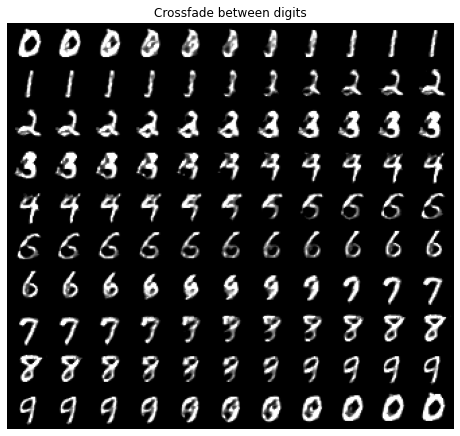Writing Numbers with a GAN¶

This notebook is an example of using Conditional Generative Adversarial Network (GANs) to generate ‘hand written’ digits.

Recognizing handwritten digits from the MNIST dataset is a common ML ‘hello world’. What about the opposite? Instead of converting an image of a handwritten digit to a number, what about converting a number to a handwritten digit?

One approach would be to train a neural network to recognize digits, then ‘drive it backwards’ and use it to synthesize images. We’ll see why that doesn’t work (after trying it out), and then demonstrate a technique called ‘Generative Adversarial Networks’ that generates realistic looking output.

In :
Collecting torch==1.4.0+cpu
Collecting torchvision==0.5.0+cpu
Collecting six (from torchvision==0.5.0+cpu)
Using cached https://files.pythonhosted.org/packages/65/eb/1f97cb97bfc2390a276969c6fae16075da282f5058082d4cb10c6c5c1dba/six-1.14.0-py2.py3-none-any.whl
Collecting numpy (from torchvision==0.5.0+cpu)
Using cached https://files.pythonhosted.org/packages/3a/5f/47e578b3ae79e2624e205445ab77a1848acdaa2929a00eeef6b16eaaeb20/numpy-1.16.6-cp27-cp27mu-manylinux1_x86_64.whl
Collecting pillow>=4.1.1 (from torchvision==0.5.0+cpu)
Installing collected packages: torch, six, numpy, pillow, torchvision
Successfully installed numpy-1.16.6 pillow-6.2.2 six-1.14.0 torch-1.4.0+cpu torchvision-0.5.0+cpu
In :
import torch
import torch.nn as nn
import torch.optim as optim
import torch.utils.data
import torchvision.datasets as datasets
import torchvision.transforms as transforms
import torchvision.utils as vutils
import numpy as np
import matplotlib.pyplot as plt
import torch.nn.functional as F
from torch.optim.lr_scheduler import StepLR

Naive Attempt: Drive a NN backwards¶

For our first attempt, we will:

1. Train a neural net to recognize handwritten digits
2. Drive it backwards.

The network to recognize digits is based on the PyTorch introductory tutorial:

In :
class SimpleDigitRecognizer(nn.Module):
"""
Recognize handwritten digits
"""
def __init__(self):
super(SimpleDigitRecognizer, self).__init__()
self.relu = nn.LeakyReLU(0.2, inplace=True)
self.conv1 = nn.Conv2d(1, 16, 3, 2, bias=False)
self.conv2 = nn.Conv2d(16, 32, 3, 2, bias=False)
self.linear1 = nn.Linear(288, 64)
self.linear2 = nn.Linear(64, 10)

def forward(self, image_of_a_digit):
X = self.relu(self.conv1(image_of_a_digit))
X = self.relu(self.conv2(X))
X = F.max_pool2d(X, 2)
X = torch.flatten(X, 1)
X = self.relu(self.linear1(X))
digit_probabilities = F.log_softmax(self.linear2(X),dim=1)
return digit_probabilities  # Not strictly probabilities

Train the network

In :
model = SimpleDigitRecognizer()
scheduler = StepLR(optimizer, step_size=1, gamma=0.7)

batch_size=64)

print_progress = False
for epoch in range(1, 15):
for batch_idx, (data, target) in enumerate(train_loader):
output = model(data)
loss = F.nll_loss(output, target)
loss.backward()
optimizer.step()
if print_progress and batch_idx % 64*64 == 0:
print('Train Epoch: {} [{}/{} ({:.0f}%)]\tLoss: {:.6f}'.format(
100. * batch_idx / len(train_loader), loss.item()))
scheduler.step()

Before we try and use model to generate digits, let's try and use it as it is intended, to recognize digits:

In :
plt.figure(figsize=(8,8))
plt.axis("off")
plt.title("Training Images")
_ = plt.imshow(np.transpose(bb,(1,2,0)))
_, guesses = model(training_images).max(dim=1)
guesses.reshape(8,8)
Out:
tensor([[5, 0, 4, 1, 9, 2, 1, 3],
[1, 4, 3, 5, 3, 6, 1, 7],
[2, 8, 6, 9, 4, 0, 9, 1],
[1, 2, 4, 3, 2, 7, 3, 8],
[6, 9, 0, 5, 6, 0, 7, 6],
[1, 8, 7, 9, 3, 9, 8, 5],
[9, 3, 3, 0, 7, 4, 9, 8],
[0, 9, 4, 1, 4, 4, 6, 0]])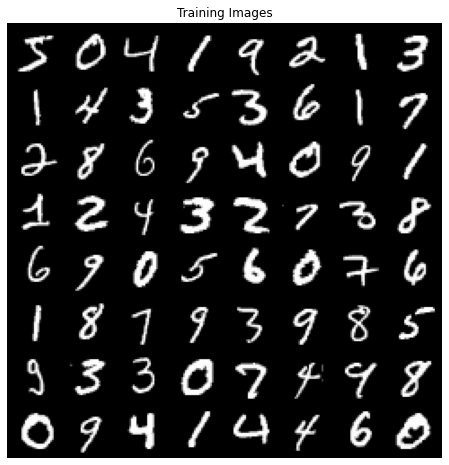We have a neural network that can recognize digits, now use PyTorch's optimizer to find an input that is recognized as the digit we want. We'll use a batch size of 10 to generate all 10 digits at once.

In :
Out:
tensor([0, 1, 2, 3, 4, 5, 6, 7, 8, 9])

input_image is the target of the optimizer. It needs the requires_grad flag so that PyTorch can keep track of how changes to it affect the output that we are optimizing.

In :
input_image = torch.full([10, 1, 28, 28], 0.5, dtype=torch.float32, requires_grad=True)

We are going to optimize the input image, without changing the trained neural network model, so input_image is the only parameter passed to the optimizer.

In :

The optimization process will modify input_image to minimize the value of loss, which is the difference between the output of the model and target answer.

In :
scheduler = StepLR(optimizer, step_size=1, gamma=0.7)
for epoch in range(1, 5):
for i in range(5000):
output = model(input_image.clamp(0.0, 1.0))
loss.backward()
optimizer.step()
# print("Epoch %d: Total Loss: %.4f" % (epoch, loss))
scheduler.step()

Let's feed those images into our model and see what we get:

In :
final_image = input_image.clamp(0.0, 1.0).detach()
_, guesses = model(final_image).max(dim=1)
print(guesses)
tensor([0, 1, 2, 3, 4, 5, 6, 7, 8, 9])

So we've found a set of images that are digits, according to the model. Let's look at them:

In :
plt.figure(figsize=(8,8))
plt.axis("off")
plt.title("Generated Digits 0..9")
bb = vutils.make_grid(final_image, padding=2, nrow=10, normalize=True)
_ = plt.imshow(np.transpose(bb,(1,2,0)))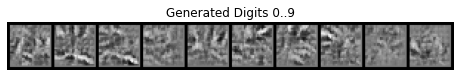They certainly don't look like digits to us!

The problem here is that the classifier divides 784-dimensional space (one dimension for each of the 28x28 pixels) into 10 categories, one for each digit. This space is large, but the classifier has only seen training examples for the parts of it that look like a number. For the parts where it hasn’t seen training data, the output is basically a random choice of the 10 digits. When we ask the optimizer to find a ‘4’ it will find an image that SimpleDigitRecognizer strongly believes is a number ‘4’, but it will turn out to be a point somewhere in untrained backwaters of this 784-dimensional space that doesn’t look like a number ‘4’ to you or I.

One alternative is a thing called a ‘Generative Adversarial Network’ or GAN.

Working solution: GAN¶

Instead of driving a classifier backwards, this sets up a system of a two separate networks called a ‘Generator’ (G) and a ‘Discriminator’ (D).

The Generator is given a random seed and told to ‘generate a number 4’. It does this without ever seeing what a human-written ‘4’ looks like.

The Discriminator takes a sample image and must determine if it is a real training image, or a 'fake' output from the Generator.

These two are trained alternately:

• The Generator learns to fool the Discriminator
• The Discriminator learns to distinguish real from fake numbers.

If there is any systematic difference between a human and the Generator, then the Discriminator will learn it. This might be anything from ‘the image is white noise’ to ‘the writing is too neat’. The Generator will then have to learn to generate things that more closely match (in a probability distribution sense) what people draw.

In :
class Discriminator(nn.Module):
def __init__(self):
super(Discriminator, self).__init__()
self.relu = nn.LeakyReLU(0.2, inplace=True)
self.conv1 = nn.Conv2d(1, 16, 3, 2, bias=False)
self.dropout1 = nn.Dropout2d()
self.conv2 = nn.Conv2d(16, 32, 3, 2, bias=False)
self.linear1 = nn.Linear(288 + 10, 64)
self.linear2 = nn.Linear(64 + 10, 1)

def forward(self, number, image):
X = self.relu(self.conv1(image))
X = self.relu(self.conv2(X))
X = F.max_pool2d(X, 2)
X = torch.flatten(X, 1)
X = torch.cat((X, number), dim=1)
X = self.relu(self.linear1(X))
X = torch.cat((X, number), dim=1)
X = torch.sigmoid(self.linear2(X))
return X

class Generator(nn.Module):
def __init__(self):
super(Generator, self).__init__()
self.relu = nn.LeakyReLU(0.2)
self.linear1 = nn.Linear(13, 7*7*16)
self.conv1 = nn.ConvTranspose2d(in_channels=16, out_channels=8, kernel_size=4,  stride=2, padding=1)
self.conv2 = nn.ConvTranspose2d(in_channels=8, out_channels=4, kernel_size=4,  stride=2, padding=1)
self.conv3 = nn.ConvTranspose2d(in_channels=4, out_channels=1, kernel_size=3,  stride=1, padding=1)

def forward(self, number_to_generate, random):
batch_size = number_to_generate.size()
X = torch.cat((number_to_generate, random), dim=1)
X = self.linear1(X)
X = torch.reshape(X, (batch_size, 16, 7,7))
X = self.relu(self.conv1(X))
X = self.relu(self.conv2(X))
X = self.relu(self.conv3(X))
X = torch.sigmoid(X) * 1.2 - 0.1
X = torch.clamp(X, 0.0, 1.0)
return X
In :
batch_size = 128
transform=transforms.Compose([
transforms.ToTensor()
])),
batch_size=batch_size,
shuffle=True,
drop_last=True)
In :
plt.figure(figsize=(8,8))
plt.axis("off")
plt.title("Sample Training Images")
_ = plt.imshow(np.transpose(bb,(1,2,0)))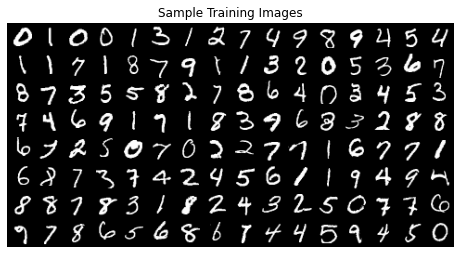Initialize the weights of the model to random values, this makes the model easier to train.

In :
def weights_init(m):
classname = m.__class__.__name__
if classname.find('Conv') != -1:
nn.init.normal_(m.weight.data, 0.0, 0.02)
elif classname.find('BatchNorm') != -1:
nn.init.normal_(m.weight.data, 1.0, 0.02)
nn.init.constant_(m.bias.data, 0)
model_g = Generator()
model_d = Discriminator()
model_g.apply(weights_init)
model_d.apply(weights_init)
None

There isn't an obvious objective way to define what 'good' output of the generator is. Our goal is that it 'looks human', so displaying a selection of generated samples is reasonable. sample_output() displays 10 samples of each of the 10 digits. By using the same input randomness each time is it easier to see progress made through training

In :
sample_output_randomness = torch.randn(100, 3)

def sample_output():
numbers = [n for n in range(0, 10) for _ in range(0,10)]
labels_one_hot = F.one_hot(torch.as_tensor(numbers), 10).to(dtype=torch.float)
fake_output = model_g.forward(labels_one_hot, sample_output_randomness)
return vutils.make_grid(fake_output, nrow=10)
In :
def train(epochs=20):
sample_outputs = []
d_loss_history = []
g_loss_history = []
scheduler_g = StepLR(optimizer_g, step_size=5, gamma=0.7)
scheduler_d = StepLR(optimizer_d, step_size=5, gamma=0.7)
criterion = nn.BCELoss()
labels_real = torch.full((batch_size, 1), 1.0)
labels_fake = torch.full((batch_size, 1), 0.0)
for epoch in range(1, epochs):
for batch_idx, (data, labels) in enumerate(train_loader):
labels_one_hot = F.one_hot(labels, 10).to(dtype=torch.float)
# Train the discriminator
d_correct = model_d.forward(labels_one_hot, data)

# Generate fake data
fake_output = model_g.forward(labels_one_hot, torch.randn(batch_size, 3))
d_fake = model_d.forward(labels_one_hot, fake_output)

err_d_real = criterion(d_correct, labels_real)
err_d_fake = criterion(d_fake, labels_fake)
err_d = err_d_real + err_d_fake
err_d.backward()
optimizer_d.step()
d_loss_history.append(err_d.item())

# Train the generator
# Generate fake data
fake_output = model_g.forward(labels_one_hot, torch.randn(batch_size, 3))
# Feed it to the discriminator
d_fake = model_d.forward(labels_one_hot, fake_output)
# The goal here is for the discriminator to think these are real
# (we are training the generator, here)
g_wins = criterion(d_fake, labels_real)
g_wins.backward()
optimizer_g.step()
g_loss_history.append(g_wins.item())

# print('epoch:%2d err_d_real:%.4f  err_d_fake:%.4f' % (epoch, err_d_real.item(), err_d_fake.item()))
output = sample_output()
# vutils.save_image(output, "output-%02d.png" % (epoch))
sample_outputs.append(output)

scheduler_g.step()
scheduler_d.step()
return sample_outputs, g_loss_history, d_loss_history

sample_outputs, g_loss_history, d_loss_history = train(20)
In :
plt.plot(g_loss_history, label="G Loss")
plt.plot(d_loss_history, label="D Loss")
plt.legend()
None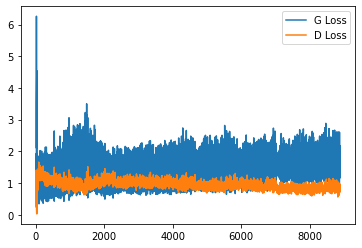In :
plt.figure(figsize=(8,8))
plt.axis("off")
plt.title("After 1 training epoch")
_ = plt.imshow(np.transpose(sample_outputs,(1,2,0)))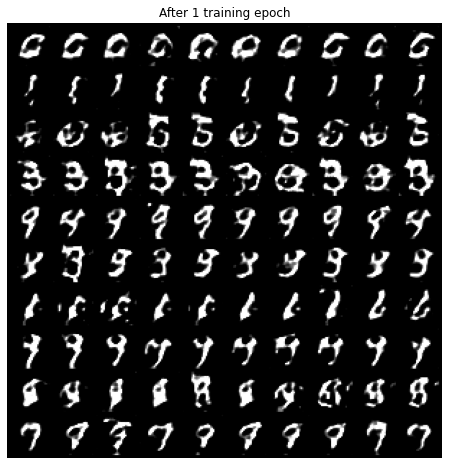In :
plt.figure(figsize=(8,8))
plt.axis("off")
plt.title("Final generated output")
_ = plt.imshow(np.transpose(sample_output(),(1,2,0)))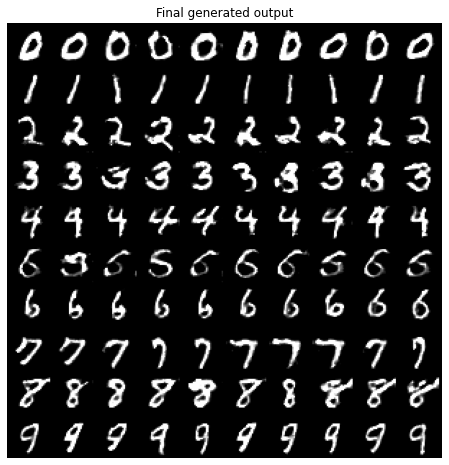Done!

Also, we can interpolate between numbers:

In :
t = torch.zeros([110, 10], dtype=torch.float32)
for i in range(10):
for p in range(11):
cell = 11*i + p
f = p/10.
t[cell, i] = 1.0 - f
t[cell, (i+1) % 10] = f